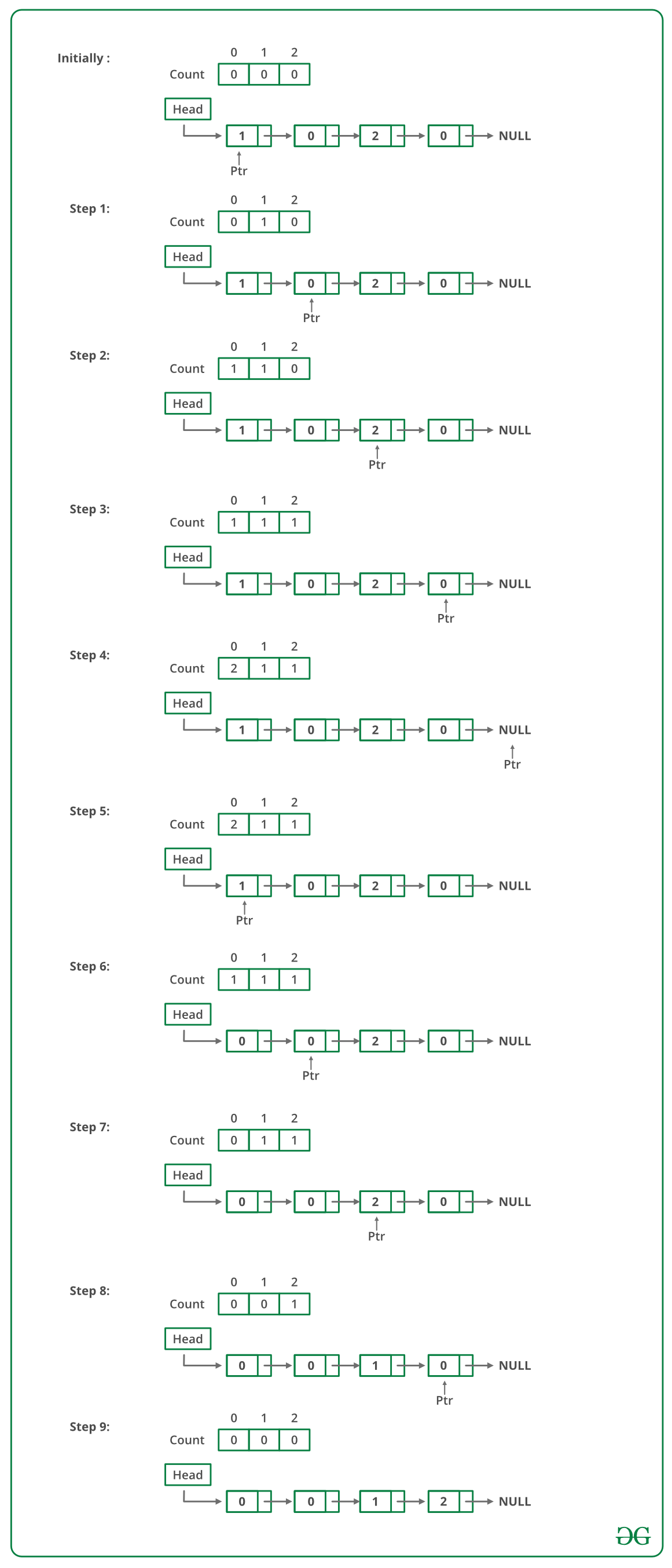Open In App

# Python Program For Sorting A Linked List Of 0s, 1s And 2s

Given a linked list of 0s, 1s and 2s, sort it.
Examples:

```Input: 1 -> 1 -> 2 -> 0 -> 2 -> 0 -> 1 -> NULL
Output: 0 -> 0 -> 1 -> 1 -> 1 -> 2 -> 2 -> NULL

Input: 1 -> 1 -> 2 -> 1 -> 0 -> NULL
Output: 0 -> 1 -> 1 -> 1 -> 2 -> NULL
```

Source: Microsoft Interview | Set 1

Following steps can be used to sort the given linked list.

• Traverse the list and count the number of 0s, 1s, and 2s. Let the counts be n1, n2, and n3 respectively.
• Traverse the list again, fill the first n1 nodes with 0, then n2 nodes with 1, and finally n3 nodes with 2.

Below image is a dry run of the above approach:Below is the implementation of the above approach:

## Python

 `# Python program to sort a linked list ``# of 0, 1 and 2``class` `LinkedList(``object``):``    ``def` `__init__(``self``):`` ` `         ``# Head of list``         ``self``.head ``=` `None`` ` `    ``# Linked list Node``    ``class` `Node(``object``):``        ``def` `__init__(``self``, d):``            ``self``.data ``=` `d``            ``self``.``next` `=` `None`` ` `    ``def` `sortList(``self``):`` ` `        ``# Initialise count of 0 1``        ``# and 2 as 0``        ``count ``=` `[``0``, ``0``, ``0``]`` ` `        ``ptr ``=` `self``.head`` ` `        ``# Count total number of '0', '1' ``        ``# and '2'``        ``# count will store total number ``        ``# of '0's``        ``# count will store total number ``        ``# of '1's``        ``# count will store total number   ``        ``# of '2's  ``        ``while` `ptr !``=` `None``:``            ``count[ptr.data] ``+``=` `1``            ``ptr ``=` `ptr.``next`` ` `        ``i ``=` `0``        ``ptr ``=` `self``.head`` ` `        ``# Let say count = n1, count = n2 ``        ``# and count = n3``        ``# now start traversing list from head node,``        ``# 1) fill the list with 0, till n1 > 0``        ``# 2) fill the list with 1, till n2 > 0``        ``# 3) fill the list with 2, till n3 > 0  ``        ``while` `ptr !``=` `None``:``            ``if` `count[i] ``=``=` `0``:``                ``i``+``=``1``            ``else``:``                ``ptr.data ``=` `i``                ``count[i] ``-``=` `1``                ``ptr ``=` `ptr.``next`` ` ` ` `    ``# Utility functions``    ``# Inserts a new Node at front of ``    ``# the list.``    ``def` `push(``self``, new_data):`` ` `        ``# 1 & 2: Allocate the Node &``        ``#        Put in the data``        ``new_node ``=` `self``.Node(new_data)`` ` `        ``# 3. Make next of new Node as head``        ``new_node.``next` `=` `self``.head`` ` `        ``# 4. Move the head to point to new Node``        ``self``.head ``=` `new_node`` ` `    ``# Function to print linked list``    ``def` `printList(``self``):``        ``temp ``=` `self``.head``        ``while` `temp !``=` `None``:``            ``print` `str``(temp.data),``            ``temp ``=` `temp.``next``        ``print` `''`` ` `# Driver code``llist ``=` `LinkedList()``llist.push(``0``)``llist.push(``1``)``llist.push(``0``)``llist.push(``2``)``llist.push(``1``)``llist.push(``1``)``llist.push(``2``)``llist.push(``1``)``llist.push(``2``)`` ` `print` `"Linked List before sorting"``llist.printList()`` ` `llist.sortList()`` ` `print` `"Linked List after sorting"``llist.printList()``# This code is contributed by BHAVYA JAIN`

Output:

```Linked List Before Sorting
2  1  2  1  1  2  0  1  0